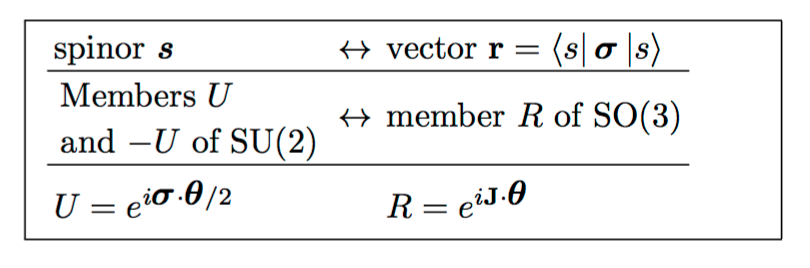# What kind of geometric object is the Pauli spin matrix vector $\vec{\sigma} = (\sigma_1, \sigma_2, \sigma_3)$?

Physicists routinely wrote all 3 Pauli spin matrices as a vector.

$$\sigma_1 = \left( \begin{array}{cc} 0 & 1 \\ 1 & 0\end{array} \right) \hspace{0.25in} \sigma_2 = \left( \begin{array}{cc} 0 & -i \\ i & 0\end{array} \right)\hspace{0.25in} \sigma_3 = \left( \begin{array}{cc} 1 & 0 \\ 0 & -1\end{array} \right)$$

This enables them to write shortcuts in quantum mechanics books such as:

$$e^{ i\theta (\vec{v} \cdot \vec{\sigma})} = (\cos \theta) I + ( i \sin \theta) (\vec{v} \cdot \vec{\sigma})$$

What kind of object is $\vec{\sigma}$ ? Is it a vector or a matrix or both?

Another example is when we write the curl as a determinant.

$$\nabla \times F = \left| \begin{array}{ccc} \mathbf{i} & \mathbf{j} & \mathbf{k} \\ \frac{\partial}{\partial x} & \frac{\partial}{\partial y} & \frac{\partial}{\partial z} \\ F_x & F_y & F_z \end{array} \right|$$

Curl is not the volume of any kind of box. Is there a rigorous geometric meaning for these "abuses of notation"?

I asked this question on Math.SE and got a very literal answer, which did not help any. So I am asking here. You are welcome to answer there and call it a day.

The Pauli spin vector $$\vec\sigma$$ relates a two-component spinor $$s={\alpha\choose \beta}$$ to its corresponding three-component vector $$\vec{v}=(a,b,c)$$. In Dirac bra-ket notation the relation is written as $$\vec{v}=\langle s|\vec\sigma|s\rangle,$$ in components $$v_i=s^\ast\sigma_i s$$. A pair of unitary operations $$\pm U\in \text{SU}(2)$$ on $$s\mapsto s'$$ is thereby mapped onto a rotation $$R\in \text{SO}(3)$$ of $$\vec{v}\mapsto \vec{v}'=\langle s'|\vec\sigma|s'\rangle$$. The mapping is illustrated by this tableThe usual vector identities do not apply to $$\vec\sigma$$, for example, instead of $$\vec a\cdot(\vec b\times\vec a)=0$$, one has $$\vec \sigma\cdot(\vec v\times\vec \sigma)=-2i\,\vec v\cdot\vec\sigma$$.
The Pauli spin vector encodes a two-complex-dimensional unitary representation of the Lie algebra $\bf{su}_2$. A basis of the Lie algebra maps to the matrix algebra $\bf{gl}_2(\mathbb{C}),$ giving a $3\times 2\times 2$ tensor. In 3 dimensions, physicists will sometimes blur the distinction between the basis for the tangent space and the Lie algebra of the Lorenz group $SU(2)$ at the origin, by the identification of $\hat{x}_i$ with the operator $\hat{x}_i\times -$ (this is not possible in higher dimensions), but these three matrices should really be thought of as parametrized by a basis for the Lie algebra.